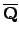##and Number Fields

has many commands for doing basic arithmetic with.
> Qbar := AlgebraicClosure(RationalField());
> Qbar;
> S<x> := PolynomialRing(Qbar);
> r := Roots(x^3-2);
> r;
[
<r1, 1>,
<r2, 1>,
<r3, 1>
]
> a := r;
> MinimalPolynomial(a);
x^3 - 2
> s := Roots(x^2-7);
> b := s;
> MinimalPolynomial(b);
x^2 - 7
> a+b;
r4 + r1
> MinimalPolynomial(a+b);
x^6 - 21*x^4 - 4*x^3 + 147*x^2 - 84*x - 339
> Trace(a+b);
0
> Norm(a+b);
-339

There are few commands for general algebraic number fields, so usually we work in specific finitely generated subfields:
> MinimalPolynomial(a+b);
x^6 - 21*x^4 - 4*x^3 + 147*x^2 - 84*x - 339
> K := NumberField($1) ; //$1 = result of previous computation.
> K;
Number Field with defining polynomial x^6 - 21*x^4 - 4*x^3 +
147*x^2 - 84*x - 339 over the Rational Field

We can also define relative extensions of number fields and pass to the corresponding absolute extension.
> R<x> := PolynomialRing(RationalField());
> K<a> := NumberField(x^3-2);   // a is the image of x in Q[x]/(x^3-2)
> a;
a
> a^3;
2
> S<y> := PolynomialRing(K);
> L<b> := NumberField(y^2-a);
> L;
Number Field with defining polynomial y^2 - a over K
> b^2;
a
> b^6;
2
> AbsoluteField(L);
Number Field with defining polynomial x^6 - 2 over the Rational
Field


William Stein 2004-05-06